TitleCollege Algebra
Tutorial 25: Slope of a LineLearning Objectives

 After completing this tutorial, you should be able to: Know when a line rises, falls, is horizontal or is vertical. Find the slope given two points on the line.Introduction

 In this tutorial we will explore the slope of the line.  Basically, the slope of the line measures the steepness of the line.  We will start with some visuals to help you understand what the slope of a straight line tells us about the line.  Next, we will practice finding the slope given two points on the line.  Let's see what you can do with slopes.Tutorial

 Slope

The slope of a line measures the steepness of the line.

Most of you are probably familiar with associating slope with "rise over run".

 Rise means how many units you move up or down from point to point.  On the graph that would be a change in the y values. Run means how far left or right you move from point to point.  On the graph, that would mean a change of x values.

Here are some visuals to help you with this definition:

Positive Slope: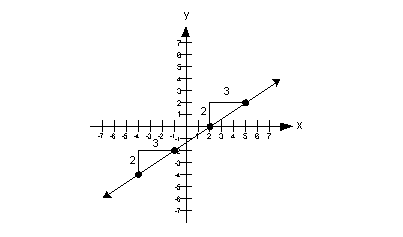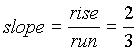Note that when a line has a positive slope it rises up left to right.

 Negative Slope: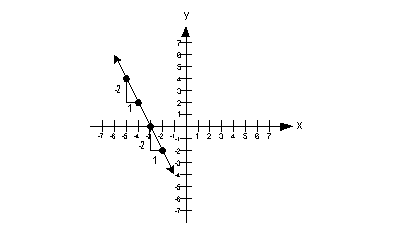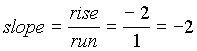Note that when a line has a negative slope it falls left to right.

 Zero Slope: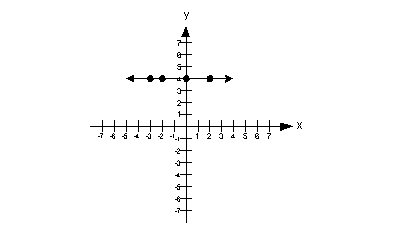slope = 0  Note that when a line is horizontal the slope is 0.

 Undefined Slope: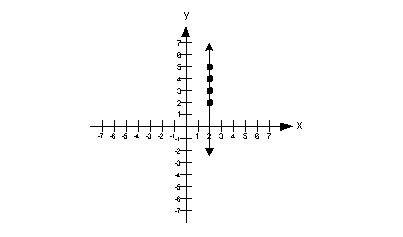slope = undefined  Note that when the line is vertical the slope is undefined.

 Slope Formula Given Two Points Given two points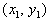and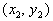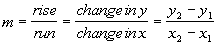The subscripts just indicate that these are two different points.  It doesn't matter which one you call point 1 and which one you call point 2 as long as you are consistent throughout that problem.  Note that we use the letter m to represent slope.Example 1: Find the slope of the straight line that passes through (7, 5) and (5, 1) or state that the slope is undefined.  Then indicate if the line through the points rises (left to right), falls (left to right), is horizontal, or is vertical.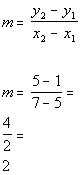*Plug in x and y values into slope formula *Simplify

 The slope of the line is 2. Since the slope is positive, the line would rise (left to right).Example 2: Find the slope of the straight line that passes through (1, -4) and (-1, 3) or state that the slope is undefined.  Then indicate if the line through the points rises (left to right), falls (left to right), is horizontal, or is vertical.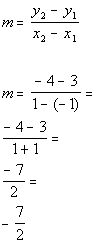*Plug in x and y values into slope formula *Simplify

 Make sure that you are careful when one of your values is negative and you have to subtract it as we did in line 2.  1 - (-1) is not the same as 1 - 1.  The slope of the line is -7/2. Since the slope is negative, the line would fall (left to right).Example 3: Find the slope of the straight line that passes through (4, 1) and (-2, 1) or state that the slope is undefined.  Then indicate if the line through the points rises (left to right), falls (left to right), is horizontal, or is vertical.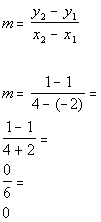*Plug in x and y values into slope formula *Simplify

 Make sure that you are careful when one of your values is negative and you have to subtract it as we did in line 2.  4 - (-2) is not the same as 4 - 2.  The slope of the line is 0. Since the slope is zero, the line would be horizontal.Example 4: Find the slope of the straight line that passes through (-2, 3) and (-2, 5) or state that the slope is undefined.  Then indicate if the line through the points rises (left to right), falls (left to right), is horizontal, or is vertical.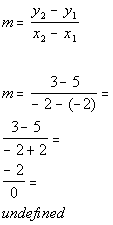*Plug in x and y values into slope formula *Simplify

 Make sure that you are careful when one of your values is negative and you have to subtract it as we did in line 2.  -2 - (-2) is not the same as -2 - 2.  The slope of the line is undefined. Since the slope is undefined, the line would be vertical.Practice Problems

 These are practice problems to help bring you to the next level.  It will allow you to check and see if you have an understanding of these types of problems. Math works just like anything else, if you want to get good at it, then you need to practice it.  Even the best athletes and musicians had help along the way and lots of practice, practice, practice, to get good at their sport or instrument.  In fact there is no such thing as too much practice. To get the most out of these, you should work the problem out on your own and then check your answer by clicking on the link for the answer/discussion for that  problem.  At the link you will find the answer as well as any steps that went into finding that answer.Practice Problems 1a - 1d: Find the slope of the straight line that passes through the given points or state that the slope is undefined.  Then indicate if the line through the points rises (left to right), falls (left to right), is horizontal, or is vertical.

 1a. (2, -5) and (-1, -1) (answer/discussion to 1a) 1b. (7, 1) and (7, 2) (answer/discussion to 1b)

 1c. (1, 7) and (-2, -2) (answer/discussion to 1c) 1d.  (-1, -5) and (-4, -5) (answer/discussion to 1d)Need Extra Help on these Topics?

The following are webpages that can assist you in the topics that were covered on this page:

 http://www.wtamu.edu/academic/anns/mps/math/mathlab/int_algebra/int_alg_tut15_slope.htm This website helps you with the slope of a line. http://www.purplemath.com/modules/slope.htm This webpage helps you with slope. http://www.math.com/school/subject2/lessons/S2U4L2DP.html This website covers slopes and y-intercept.

Go to Get Help Outside the Classroom found in Tutorial 1: How to Succeed in a Math Class for some more suggestions.

Videos at this site were created and produced by Kim Seward and Virginia Williams Trice.
Last revised on Jan. 16, 2010 by Kim Seward.
All contents copyright (C) 2002 - 2010, WTAMU and Kim Seward. All rights reserved.